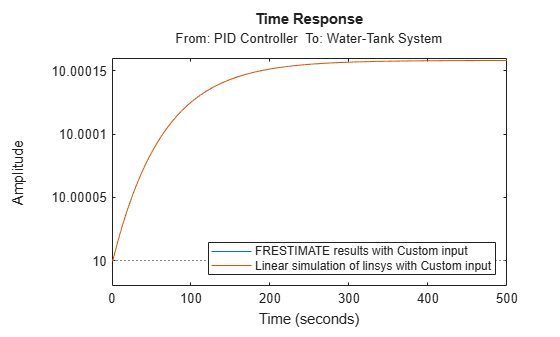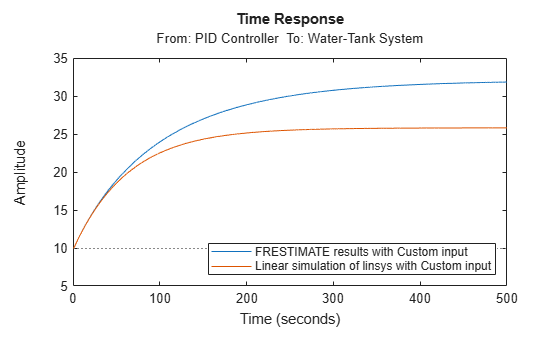# Validate Linearization in Time Domain

This example shows how to validate linearization results by comparing the simulated output of the nonlinear model and the linearized model.

```sys = 'watertank'; load_system(sys) sys_io(1) = linio('watertank/PID Controller',1,'input'); sys_io(2) = linio('watertank/Water-Tank System',1,'openoutput'); opspec = operspec(sys); op = findop(sys,opspec,findopOptions('DisplayReport','off')); linsys = linearize(sys,op,sys_io);```

If you linearized your model in Model Linearizer, you must export the linear model to the MATLAB® workspace. To do so in the Linear Analysis Workspace, right-click the model and select Export to MATLAB Workspace.

For time-domain validation of linearization, use `frest.createStep` to create a step signal.

```input = frest.createStep(... 'Ts',0.1,... 'StepTime',1,... 'StepSize',1e-5,... 'FinalTime',500);```

Simulate the Simulink model using the input signal. `simout` is the simulated output of the nonlinear model.

`[~,simout] = frestimate(sys,op,sys_io,input);`

Simulate the linear model `sys`, and compare the time-domain responses of the linear and nonlinear Simulink model. The step response of the nonlinear model and linearized model are close, which validates that the linearization is accurate.

```frest.simCompare(simout,linsys,input) legend('FRESTIMATE results with Custom input',... 'Linear simulation of linsys with Custom input',... 'Location','SouthEast')```Increase the amplitude of the step signal from `1.0e-5` to `1`.

```input = frest.createStep(... 'Ts',0.1,... 'StepTime',1,... 'StepSize',1,... 'FinalTime',500);```

Repeat the frequency response estimation with the increased amplitude of the input signal, and compare this time response plot to the exact linearization results.

`[~,simout2] = frestimate(sys,op,sys_io,input);`

Using `frest.simCompare`, compare this time response plot to the exact linearization results. The step response of linear system you obtained using exact linearization does not match the step response of the estimated frequency response with large input signal amplitude. The linear model obtained using exact linearization does not match the full nonlinear model at amplitudes large enough to deviate from the specified operating point.

```frest.simCompare(simout2,linsys,input) legend('FRESTIMATE results with Custom input',... 'Linear simulation of linsys with Custom input',... 'Location','SouthEast')```